# Calculate the percent composition of aluminum oxide. a. 47.1% AI, 52.9% O b. 52.9% AI, 47.1%...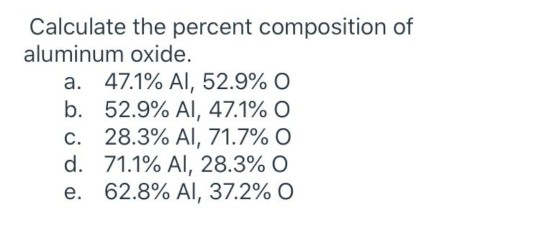Calculate the percent composition of aluminum oxide. a. 47.1% AI, 52.9% O b. 52.9% AI, 47.1% O c. 28.3% AI, 71.7% 0 d. 71.1% AI, 28.3%O e. 62.8% AI, 37.2% 0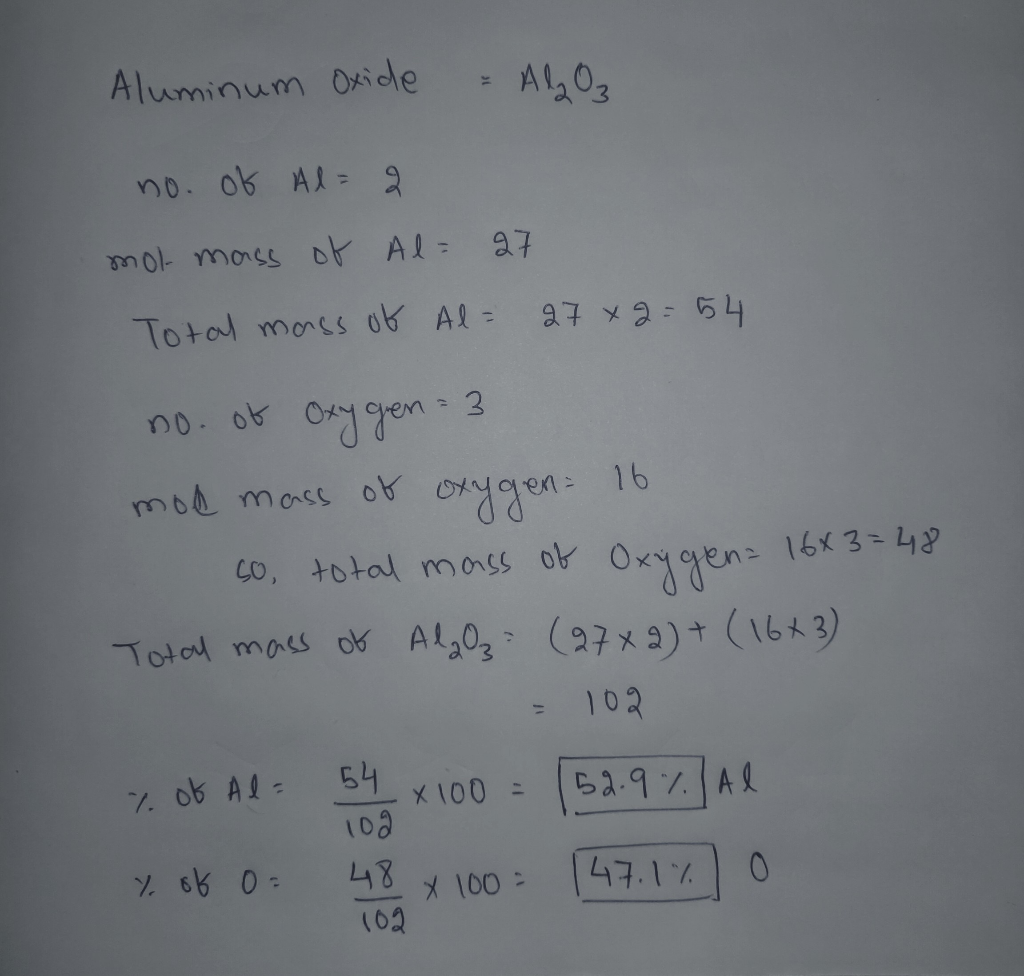#### Earn Coin

Coins can be redeemed for fabulous gifts.

Similar Homework Help Questions
• ### 9. Aluminum oxide has a composition of 52.9% aluminum and 47.1% oxygen by mass. If 19.9...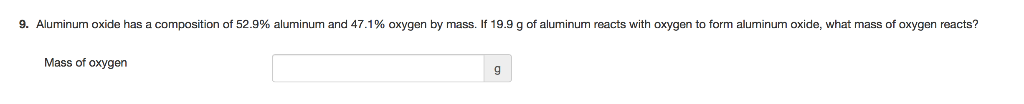9. Aluminum oxide has a composition of 52.9% aluminum and 47.1% oxygen by mass. If 19.9 g of aluminum reacts with oxygen to form aluminum oxide, what mass of oxygen reacts? Mass of oxygen 9

• ### 22. Consider the reaction: solid aluminum and oxygen gas react to form solid aluminum oxide. a....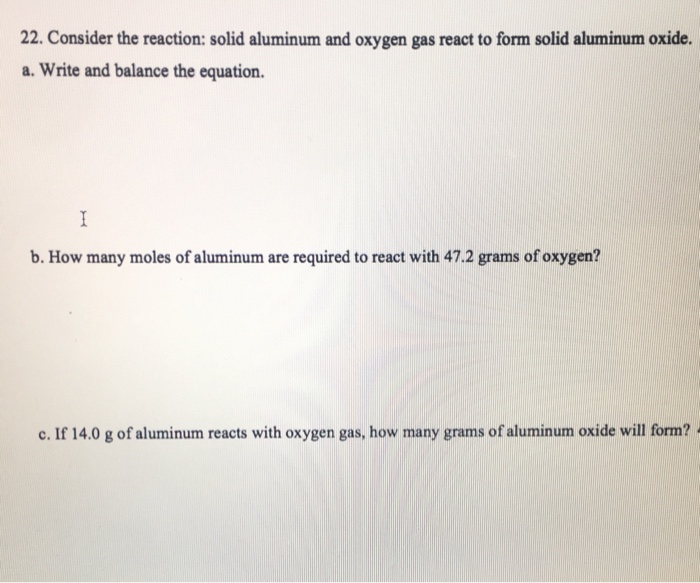22. Consider the reaction: solid aluminum and oxygen gas react to form solid aluminum oxide. a. Write and balance the equation. b. How many moles of aluminum are required to react with 47.2 grams of oxygen? c. If 14.0 g of aluminum reacts with oxygen gas, how many grams of aluminum oxide will form? d. How many atoms of aluminum are started with in part c? e. If only 19.7 g of aluminum oxide formed in the reaction from part...

• ### calculate the percent composition by mass of aluminum hydroxide AL(OH)3 M ynt (in g) of nitrogen...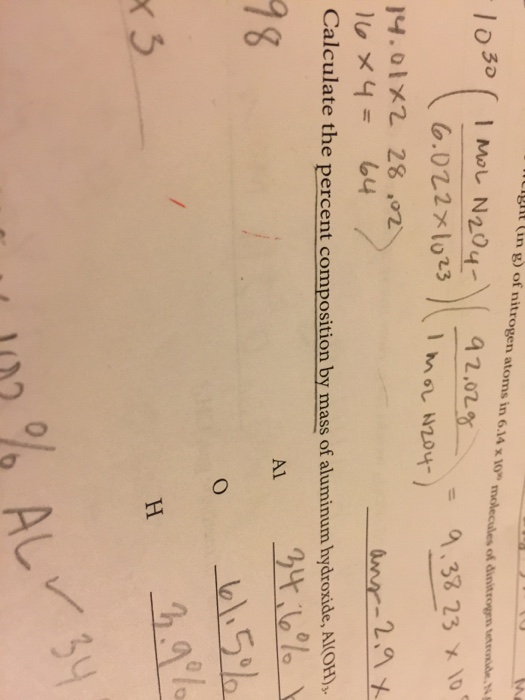calculate the percent composition by mass of aluminum hydroxide AL(OH)3 M ynt (in g) of nitrogen atoms in 6.14 x 10" molecules of dinitrogen anden 9 2.02g Imor 2204-) -1030 ( IMEL N204-) 16.022x1023 = 9.38 23 x 100 14.01X2 28.02 16*4= 64 Calculate the percent composition by mass of aluminum hydroxide, Al(OH) 34:6% ! anr-2,9x Α1 18 61.5% 3.9% x 3 102% ALY

• ### How many atoms of aluminum can be produced by the decomposition of 47.1 g of Al2O3

How many atoms of aluminum can be produced by the decomposition of 47.1 g of Al2O3? (Hint: Write and balance the equation first.) The products are oxygen gas and aluminum metal (Al) A) 1.11 X 10^24 Al atoms B) None of these C) 1.39 X 10^23 Al atoms D) 5.56 X 10^23 Al atoms E) 2.78 X 10^23 Al atoms

• ### Lean 1 Learning Objective: 7J Distinguish between actual and theoretical yield to calculate percent yield Question...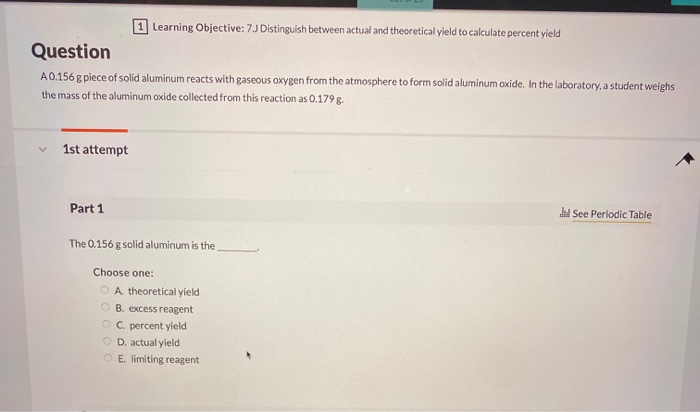Lean 1 Learning Objective: 7J Distinguish between actual and theoretical yield to calculate percent yield Question A0.156 g piece of solid aluminum reacts with gaseous oxygen from the atmosphere to form solid aluminum oxide. In the laboratory, a student weighs the mass of the aluminum oxide collected from this reaction as 0.1798- 1st attempt Part 1 See Periodic Table The 0.156 g solid aluminum is the Choose one: A theoretical yield B. excess reagent C. percent yield D. actual yield...

• ### 5. Calculate the mass of oxý gen in a 69.5 g sample of aluminum oxide. 6....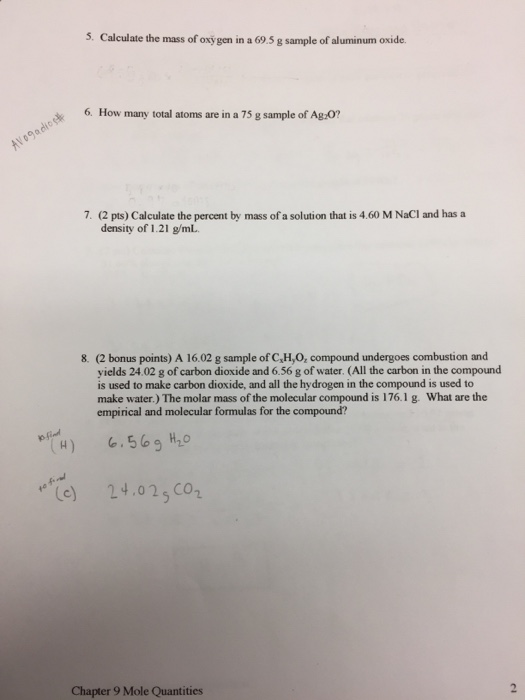5. Calculate the mass of oxý gen in a 69.5 g sample of aluminum oxide. 6. How many total atoms are in a 75 g sample of Ag:0 7. (2 pts) Calculate the percent by mass of a solution that is 4.60 M NaCI and has a density of 1.21 g/mL 8. (2 bonus points) A 16.02 g sample of C,H,O, compound undergoes combustion and yields 24.02 g of carbon dioxide and 6.56 g of water. (All the carbon in...

• ### 7.39 Calculate the mass percent composition of each of the following: a. MgF2, magnesium fluoride b....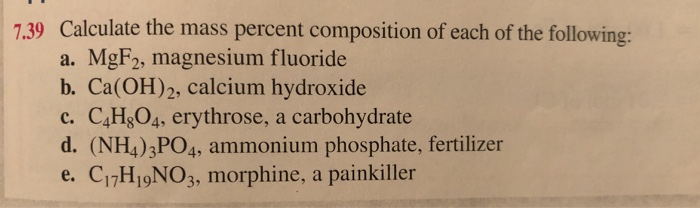7.39 Calculate the mass percent composition of each of the following: a. MgF2, magnesium fluoride b. Ca(OH)2, calcium hydroxide c. C4H204, erythrose, a carbohydrate d. (NH4)3PO4, ammonium phosphate, fertilizer e. C17H 9NO3, morphine, a painkiller

• ### A materials scientist has created an alloy containing aluminum, copper, and zinc, and wants to determine the percent composition of the alloy. The scientist takes a 12.502 g sample of the alloy a...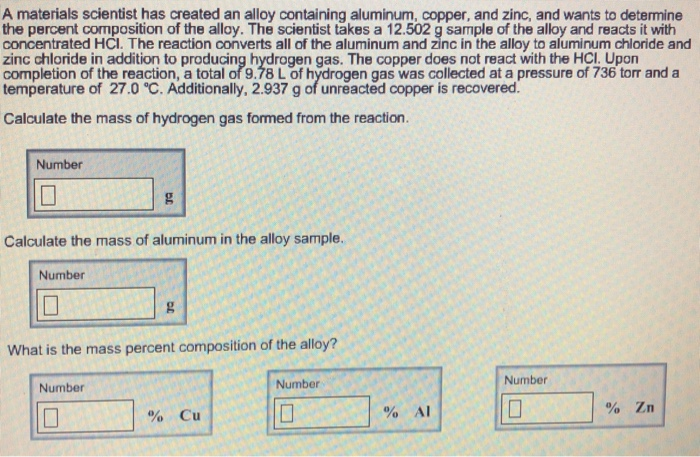A materials scientist has created an alloy containing aluminum, copper, and zinc, and wants to determine the percent composition of the alloy. The scientist takes a 12.502 g sample of the alloy and reacts it with concentrated HCI. The reaction converts all of the aluminum and zinc in the alloy to aluminum chloride and zinc chloride in addition to producing hydrogen gas. The copper does not react with the HCI. Upon completion of the reaction, a total of 9.78 L...

• ### A 0.191g piece of solid aluminum reacts with gaseous oxygen from the atmosphere to form solid...

A 0.191g piece of solid aluminum reacts with gaseous oxygen from the atmosphere to form solid aluminum oxide. In the laboratory, a student weighs the mass of the aluminum oxide collected from this reaction as 0.199 g. The 0.191g solid aluminum is the _____. A) percent yield, B) excess reagent, C) limiting reagent, D) actual yield, E) theoretical yield The 0.199g aluminum oxide is the ______. A) actual yield, B) percent yield, C) limiting reagent, D) theoretical yield, E) excess...

• ### 9.25 Calculate the mass percent composition (percent by mass of each element) in the following compounds....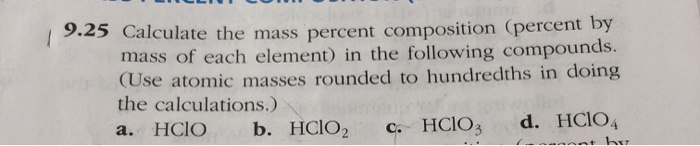9.25 Calculate the mass percent composition (percent by mass of each element) in the following compounds. (Use atomic masses rounded to hundredths in doing the calculations.) a. HCIO b. HClO2 c. HCIO3 d. HCIO ( the